StatLect

# Linear span

The span of a set of vectors, also called linear span, is the linear space formed by all the vectors that can be written as linear combinations of the vectors belonging to the given set.## Definition

Let us start with a formal definition of span.

Definition Letbe a linear space. Letbevectors. The linear span of, denoted by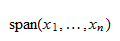is the set of all the linear combinations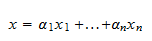that can be obtained by arbitrarily choosingscalars, ...,.

A very simple example of a linear span follows.

Example Letandbecolumn vectors defined as follows: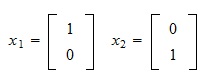Letbe a linear combination ofandwith coefficientsand. Then,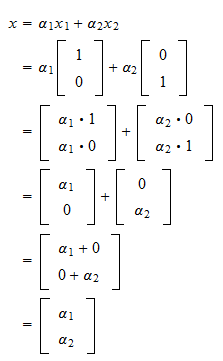Thus, the linear span is the set of all vectorsthat can be written as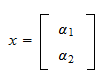whereandare two arbitrary scalars.

## A linear span is a linear space

The following proposition, although elementary, is extremely important.

Proposition The linear span of a set of vectors is a linear space.

Proof

Letbe the linear span ofvectors. Then,is the set of all vectorsthat can be represented as linear combinationsTake two vectorsandbelonging to. Then, there exist coefficientsandsuch that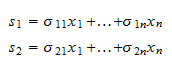The spanis a linear space if and only if, for any two coefficientsand, the linear combinationalso belongs to. But,Thus, the linear combinationcan itself be expressed as a linear combination of the vectorswith coefficients, ...,. As a consequence, it belongs to the span. In summary, we have proved that any linear combination of vectors belonging to the spanalso belongs to the span. This means thatis a linear space.

## Solved exercises

Below you can find some exercises with explained solutions.

### Exercise 1

Define the followingvectors: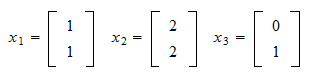Doesbelong to the linear span ofand?

Solution

The linear span ofandis the set of all vectorsthat can be written as linear combinations ofandwith scalar coefficientsand: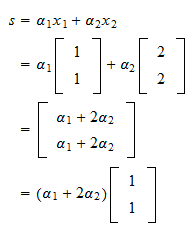In other words,contains all the scalar multiples of the vectorButis not a scalar multiple of. Therefore,does not belong to.

### Exercise 2

Does the zero vectorbelong to the span of the vectorsanddefined above?

Solution

We have proved that the span is a linear space, and the zero vector always belongs to a linear space (by the very definition of linear space).